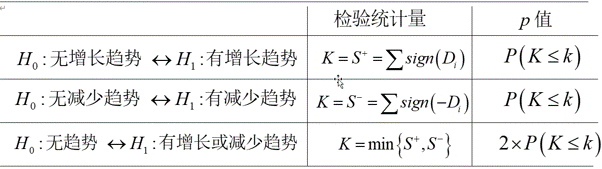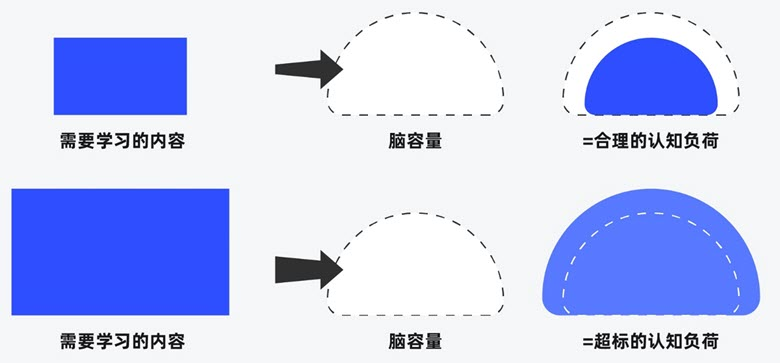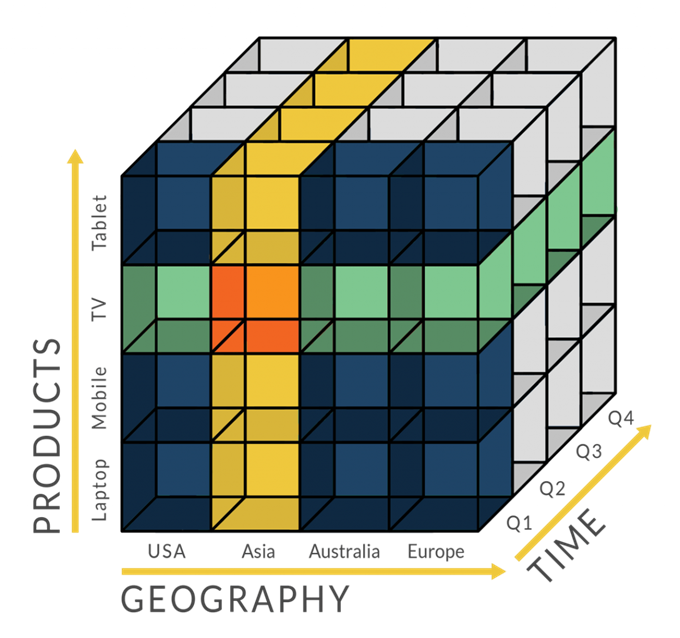# 时间序列分析之趋势判断

## 方案二：斜率法

• k > 0.1763 表示上升
• k < -0.1763 表示下降
• 其他，则表示平稳或震荡

import numpy as np
def trendline(index,data, order=1):
coeffs = np.polyfit(index, list(data), order)
slope = coeffs[-2]
return float(slope)

index=[1,2,3,4]
List=[1043,6582,5452,7571]
resultent=trendline(index,List)
print(resultent)


## 方案三：Cox-Stuart趋势检验

Cox-Stuart是一种不依赖趋势结构的快速判断趋势是否存在的方法。Cox-Stuart趋势存在性检验的理论基础是符号检验。它的检验思想是：直接考虑数据的变化趋势，若数据有上升趋势，那么排在后面的数据的值要比排在前面的数据的值显著的大，反之，若数据有下降趋势，那么排在后面的数据的值要比排在前面的数据的值显著的小，利用前后两个时期不同数据的差值正负来判断数据总的变化趋势。为保证数对同分布，前后两个数的间隔应固定。这就意味着将数据一分为二，自然形成前后数对。Cox-Staut提出最优的拆分点是数列中位于中间位置的数。

• 取$x_i$和$x_{i+c}$组成一对$(x_i, x_{i+c})$。这里如果n为偶数，则$c=n/2$，如果n是奇数，则c=(n+1)/2。当n为偶数时，共有n’=c对，而n是奇数时，共有 n’=c-1对。
• 用每一对的两元素差$D_i= x_i-x_{i+c}$的符号来衡量增减。令$S^+$为正的$D_i$的数目，$S^-$为负的$D_i$的数目。显然当正号太对时有下降趋势，反之有增长趋势。在没有趋势的零假设下他们因服从二项分布b(n’,0.5)。
• 用p(+)表示取到正数的概率，用p(-)表示取到负数的概率，这样我们就得到符号检验方法来检验序列是否存在趋势性。
 双侧检验 H0：无趋势 H1：有增长或减少趋势 左侧检验 H0：无减少趋势 H1：有减少趋势 右侧检验 H0：无增加趋势 H1：有增加趋势import scipy.stats as stats
def cos_staut(list_c,debug=False):
lst=list_c.copy()
raw_len=len(lst)
if raw_len%2==1:
del lst[int((raw_len-1)/2)]
c=int(len(lst)/2)
n_pos=n_neg=0
for i in range(c):
diff=lst[i+c]-lst[i]
if diff>0:
n_pos+=1
elif diff<0:
n_neg+=1
else:
continue
num=n_pos+n_neg
k=min(n_pos,n_neg)
p_value=2*stats.binom.cdf(k,num,0.5)
if debug:
print('fall:%i, rise:%i, p-value:%f'%(n_neg, n_pos, p_value))
if n_pos>n_neg and p_value<0.05:
return 'increasing'
elif n_neg>n_pos and p_value<0.05:
return 'decreasing'
else:
return 'no trend'

## 方案四：Mann-Kendall趋势检验法

Mann-Kendall(MK)检验(test)(Mann 1945, Kendall 1975, Gilbert 1987) 的目的是统计评估我们所感兴趣的变量，随着时间变化，是否有单调上升或下降的趋势。单调上升（下降）的趋势意味着该变量随时间增加（减少），但此趋势可能是、也可能不是线性的。MK test可替代参数线性回归分析——线性回归可检验线性拟合直线的斜率是否不为零。回归分析要求拟合回归线的残差是正态分布的，MK检验不需要这种假设，MK检验是非参数检验（不要求服从任何分布-distribution free）

• 当没有趋势时，随时间获得的数据是独立同分布的。独立的假设是说数据随着时间不是连续相关的。
• 所获得的时间序列上的数据代表了采样时的真是条件。（样本具有代表性）
• 样本的采集、处理和测量方法提供了总体样本中的无偏且具有代表性的观测值。

MK检验不要求数据是正态分布，也不要求变化趋势——如果存在的话——是线性的。如果有缺失值或者值低于一个或多个检测限制，是可以计算MK检测的，但检测性能会受到不利影响。独立性假设要求样本之间的时间足够大，这样在不同时间收集的测量值之间不存在相关性。

MK检验是检验是否拒绝零假设(null hypothesis: $H_0$)，并接受替代假设（alternative hypothesis: $H_a$）：

• $H_0$：没有单调趋势
• $H_a$：存在单调趋势

• 将数据按采集时间列出：$x_1,x_2,…,x_n$，即分别在时间1,2,…,n得到的数据。
• 确定所有n(n-1)/2个$x_j-x_k$差值的符号，其中j > k
• 令$sgn(x_j-x_k)$作为指示函数，依据$x_j-x_k$的正负号取值为1,0或-1
• 计算$S = \sum_{k-1}^{n-1}\sum_{j-k+1}^{n}sgn(x_j-x_k)$。即差值为正的数量减去差值为负的数量。如果S是一个正数，那么后一部分的观测值相比之前的观测值会趋向于变大；如果S是一个负数，那么后一部分的观测值相比之前的观测值会趋向于变小。
• 如果$n\leq 10$，依据Gilbert (1987, page 209, Section 16.4.1)中所描述，要在概率表 (Gilbert 1987, Table A18, page 272) 中查找S。如果此概率小于$\alpha$(认为没有趋势时的截止概率)，那就拒绝零假设，认为趋势存在。如果在概率表中找不到n(存在结数据——tied data values——会发生此情况)，就用表中远离0的下一个值。比如S=12，如果概率表中没有S=12，那么就用S=13来处理也是一样的。如果n > 10，则依以下步骤6-10来判断有无趋势。这里遵循的是Gilbert (1987, page 211, Section 16.4.2)中的程序。
• 计算S的方差如下：$\text{VAR}(S)=\frac{1}{18}[n(n-1)(2n+5)-\sum_{p-1}^{g}t_p(t_p-1)(2t_p+5)]$。其中g是结组（tied groups）的数量，$t_p$是第p组的观测值的数量。例如：在观测值的时间序列{23, 24, 29, 6, 29, 24, 24, 29, 23}中有g = 3个结组，相应地，对于结值(tiied value)23有$t_1= 2$、结值24有$t_2=3$、结值29有$t_3=S3$。当因为有相等值或未检测到而出现结时，VAR(S)可以通过Helsel (2005, p. 191)中的结修正方法来调整。
• 计算MK检验统计量Z_{MK}:

$$Z_{M K}=\left\{\begin{array}{cl}{\frac{S-1}{\sqrt{V A R(S)}},} & {S>0} \\{0} & {,\quad S=0} \\{\frac{S+1}{\sqrt{V A R(S)}},} & {S<0}\end{array}\right.$$

• 设想我们要测试零假设。$H_0$(没有单调趋势)对比替代假设$H_a$(有单调增趋势)，其1型错误率为$\alpha$，$0<\alpha<0.50$（注意$\alpha$是MK检验错误地拒绝了零假设时可容忍的概率——即MK检验拒绝了零假设是错误地，但这个事情发生概率是$\alpha$，我们可以容忍这个错误）。如果$Z_{MK}\geq Z_{1-\alpha}$，就拒绝零假设$H_0$，接受替代假设$H_a$，其中$Z_{1-\alpha}$是标准正态分布的$100(1-\alpha)^{th}$百分位。
• 测试上面的$H_0$与$H_a$（有单调递减趋势），其1型错误率为$alpha$，$0<\alpha<0.5$，如果$Z_{MK}\leq – Z_{1-\alpha}$，就拒绝零假设$H_0$，接受替代假设$H_a$
• 测试上面的$H_0$与$H_a$（有单调递增或递减趋势），其1型错误率为$alpha$，$0<\alpha<0.5$，如果$|Z_{MK}|\geq Z_{1-\frac{\alpha}{2}}$，就拒绝零假设$H_0$，接受替代假设$H_a$，其中竖线代表绝对值。
import math
from scipy.stats import norm, mstats
def mk_test(x, alpha=0.05):
"""
This function is derived from code originally posted by Sat Kumar Tomer
(satkumartomer@gmail.com)
The purpose of the Mann-Kendall (MK) test (Mann 1945, Kendall 1975, Gilbert
1987) is to statistically assess if there is a monotonic upward or downward
trend of the variable of interest over time. A monotonic upward (downward)
trend means that the variable consistently increases (decreases) through
time, but the trend may or may not be linear. The MK test can be used in
place of a parametric linear regression analysis, which can be used to test
if the slope of the estimated linear regression line is different from
zero. The regression analysis requires that the residuals from the fitted
regression line be normally distributed; an assumption not required by the
MK test, that is, the MK test is a non-parametric (distribution-free) test.
Hirsch, Slack and Smith (1982, page 107) indicate that the MK test is best
viewed as an exploratory analysis and is most appropriately used to
identify stations where changes are significant or of large magnitude and
to quantify these findings.
Input:
x:   a vector of data
alpha: significance level (0.05 default)
Output:
trend: tells the trend (increasing, decreasing or no trend)
h: True (if trend is present) or False (if trend is absence)
p: p value of the significance test
z: normalized test statistics
Examples
--------
>>> x = np.random.rand(100)
>>> trend,h,p,z = mk_test(x,0.05)
"""
n = len(x)

# calculate S
s = 0
for k in range(n-1):
for j in range(k+1, n):
s += np.sign(x[j] - x[k])

# calculate the unique data
unique_x, tp = np.unique(x, return_counts=True)
g = len(unique_x)

# calculate the var(s)
if n == g:  # there is no tie
var_s = (n*(n-1)*(2*n+5))/18
else:  # there are some ties in data
var_s = (n*(n-1)*(2*n+5) - np.sum(tp*(tp-1)*(2*tp+5)))/18

if s > 0:
z = (s - 1)/np.sqrt(var_s)
elif s < 0:
z = (s + 1)/np.sqrt(var_s)
else: # s == 0:
z = 0

# calculate the p_value
p = 2*(1-norm.cdf(abs(z)))  # two tail test
h = abs(z) > norm.ppf(1-alpha/2)

if (z < 0) and h:
trend = 'decreasing'
elif (z > 0) and h:
trend = 'increasing'
else:
trend = 'no trend'

return trend##### 界面设计和游戏设计中的认知负荷##### 理解认知偏差## 4 Replies to “时间序列分析之趋势判断”

1. Ding Zhaohai说道：

序列的趋势判断，看上去是个简单地不能再简单地需求，不过像通过稳健的方法对陌生的序列进行判断，需要有强大的理论支撑。

2. Ding Zhaohai说道：

最后一段代码：
“h = abs(z) > norm.ppf(1-alpha/2)”，是否出错了。
修改意见：“h = abs(z) > norm.ppf((1-alpha)/2)”

3. Ding Zhaohai说道：

是公式错误，不是代码错误。

4. Xuance说道：

Cox-Stuart趋势检验中，单边检验p_value是否不用*2
p_value=2*stats.binom.cdf(k,num,0.5)